# hi i need answer from part d Question 2 (48 marks) Consider a firm which produces a good, y, using two factors of...

hi i need answer from part d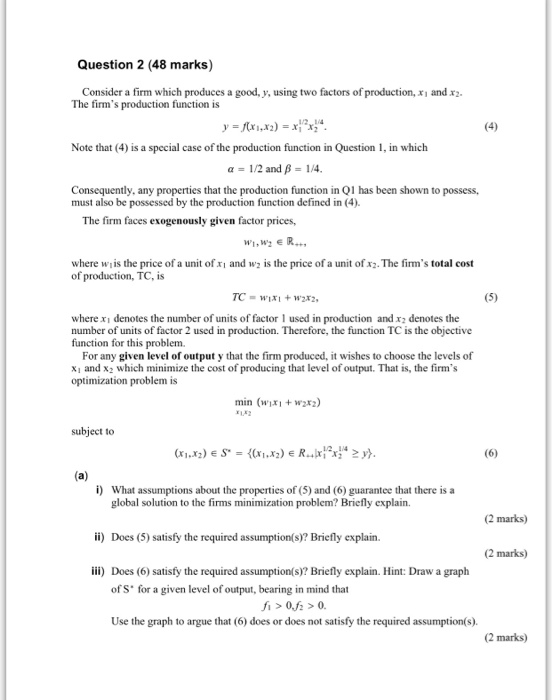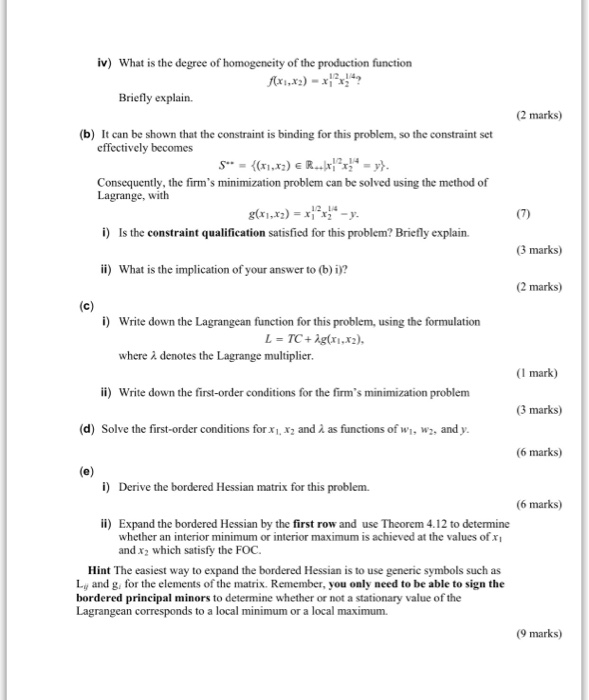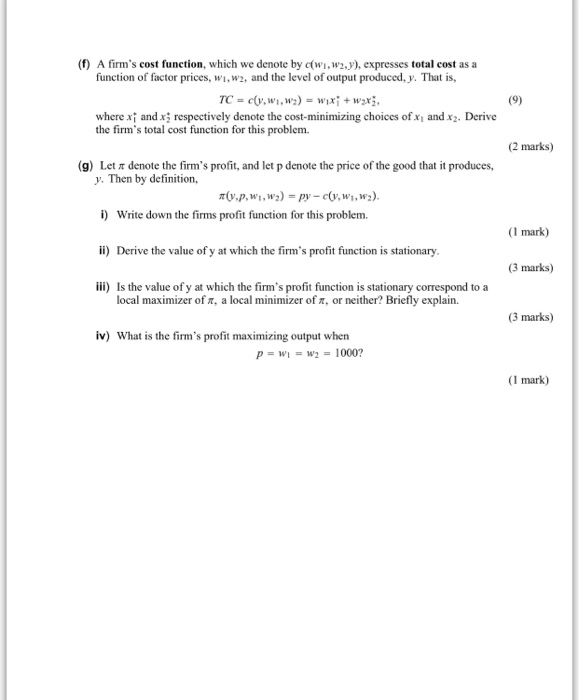Question 2 (48 marks) Consider a firm which produces a good, y, using two factors of production, xi and x2 The firm's production function is Note that (4) is a special case of the production function in Question 1, in which α-1/2 and β-14. Consequently, any properties that the production function in Q1 has been shown to possess, must also be possessed by the production function defined in (4). The firm faces exogenously given factor prices, where w is the price of a unit of xi and w is the price of a unit of x2. The firm's total cost of production, TC, is where xi denotes the number of units of factor 1 used in production and x2 denotes the number of units of factor 2 used in production. Therefore, the function TC is the objective function for this problem. For any given level of output y that the firm produced, it wishes to choose the levels of x1 and x2 which minimize the cost of producing that level of output. That is, the firm's optimization problem is min (wx +w2xz) subject to i) What assumptions about the properties of (5) and (6) guarantee that there is a global solution to the firms minimization problem? Briefly explain. (2 marks) ii) Does (5) satisfy the required assumption(s)? Briefly explain. (2 marks) ili) Does (6) satisfy the required assumption(s)? Briefly explain. Hint: Draw a graph of S' for a given level of output, bearing in mind that 0.f0. Use the graph to argue that (6) does or does not satisfy the required assumption(s) (2 marks)
iv) What is the degree of ty of the production function Briefly explain. (2 marks) (b) It can be shown that the constraint is binding for this problem, so the constraint set effectively becomes Consequently, the firm's minimization problem can be solved using the method of Lagrange, with 12 14 i) Is the constraint qualification satisfied for this problem? Briefly explain (3 marks) i) What is the implication of your answer to (b) i? (2 marks) i) Write down the Lagrangean function for this problem, using the formulation where λ denotes the Lagrange multiplier (1 mark) i) Write down the first-order conditions for the firm's minimization problem (3 marks) (d) Solve the first-order conditions for x. x2 and λ as functions of wl, w2, and y. 6 marks) i) Derive the bordered Hessian matrix for this problem. (6 marks) ii) Expand the bordered Hessian by the first row and use Theorem 4.12 to determine whether an interior minimum or interior maximum is achieved at the values of xI and x2 which satisfy the FOC. Hint The easiest way to expand the bordered Hessian is to use generic symbols such as Liy and g, for the elements of the matrix. Remember, you only need to be able to sign the bordered principal minors to determine whether or not a stationary value of the Lagrangean corresponds to a local minimum or a local maximum. (9 marks)
(f) A firm's cost function, which we denote by c(wi, w2y), expresses total cost as a function of factor prices, wi,w2, and the level of output produced, y. That is where xi and x respectively denote the cost-minimizing choices of xi and xz. Derive the firm's total cost function for this problem. (2 marks) (g) Let π denote the firm's profit, and let p denote the price of the good that it produces, y. Then by definition Write down the firms profit function for this problem. (I mark) ii) Derive the value of y at which the firm's profit function is stationary 3 marks) iii) Is the value of y at which the firm's profit function is stationary correspond to a local maximizer of π, a local minimizer or, or neither? Briefly explain. 3 marks) iv) What is the firm's profit maximizing output when (I mark)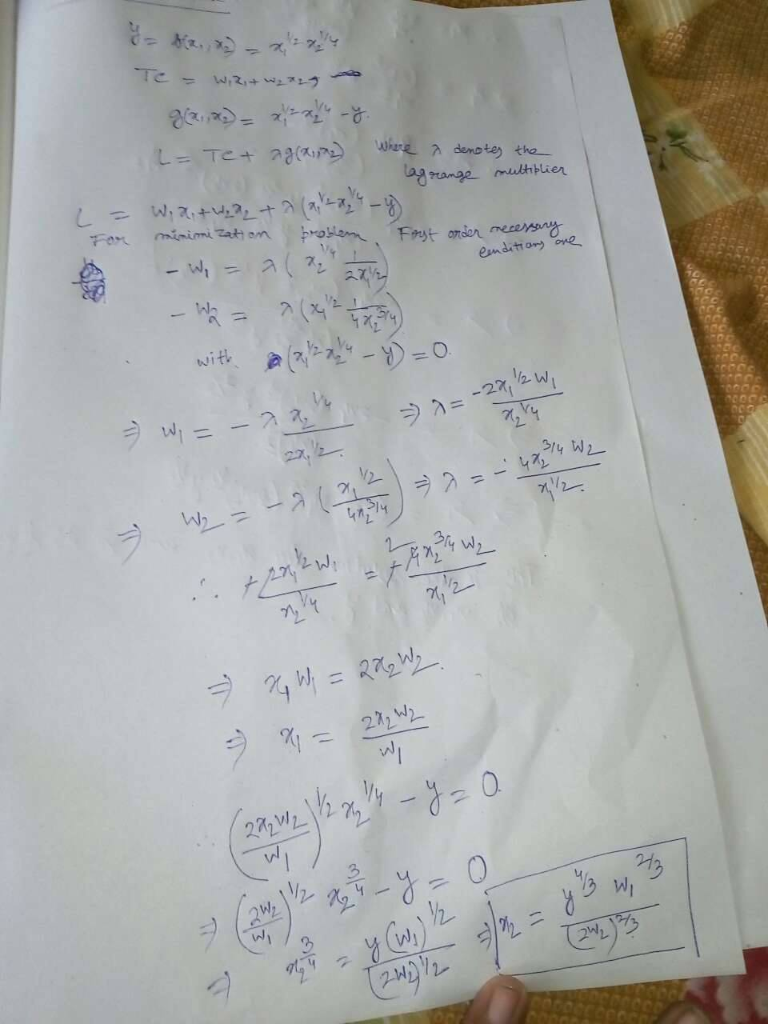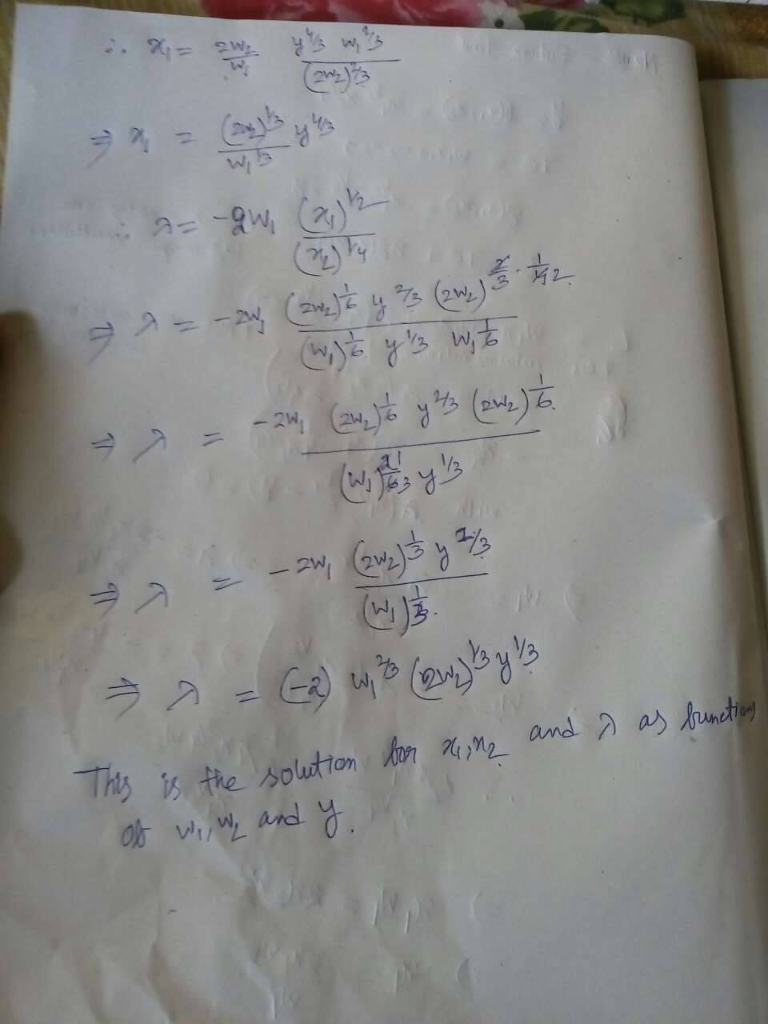##### Add Answer of: hi i need answer from part d Question 2 (48 marks) Consider a firm which produces a good, y, using two factors of...
Similar Homework Help Questions
• ### Consider a firm which produces a good, y, using two factors of production, xi and x2. The firm's production functio...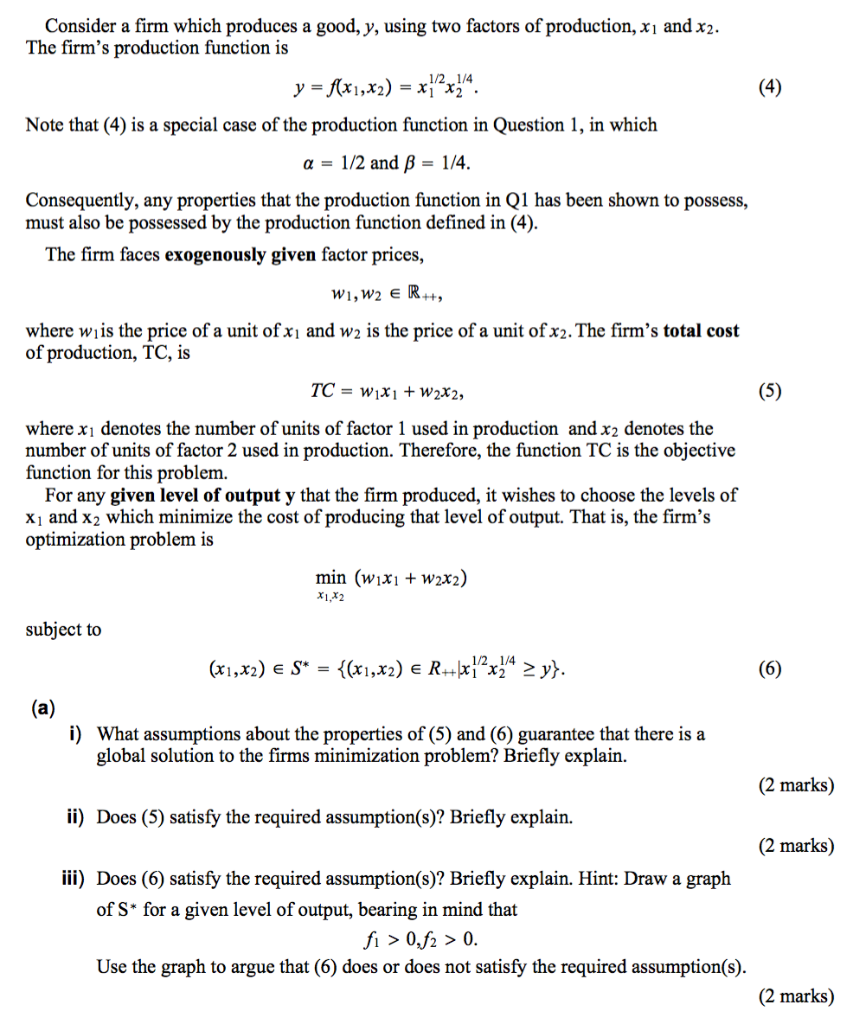Consider a firm which produces a good, y, using two factors of production, xi and x2. The firm's production function is 1/2 1/4 = xi X2. (4) Note that (4) is a special case of the production function in Question 1, in which 1/4 1/2 and B a = Consequently, any properties that the production function in Q1 has been shown to possess, must also be possessed by the production function defined in (4). The firm faces exogenously given factor...

• ### Question 1 (32 marks) Consider a firm which produces a good, y, using two inputs or factors of production, x1 and...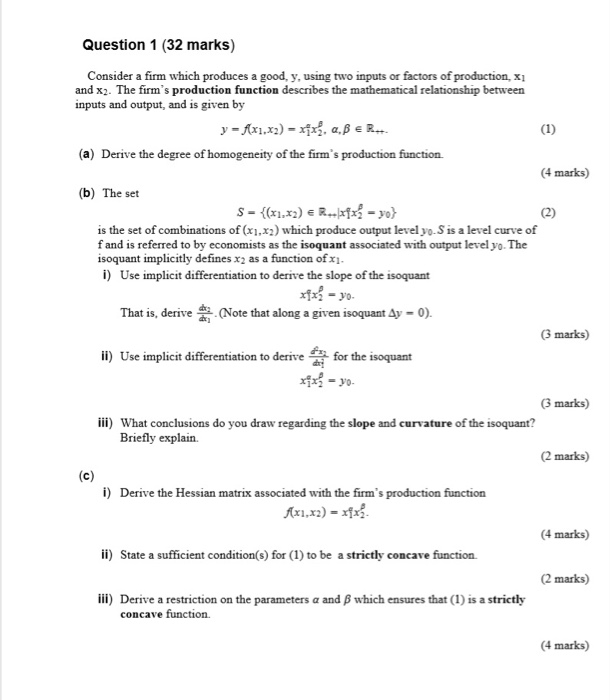Question 1 (32 marks) Consider a firm which produces a good, y, using two inputs or factors of production, x1 and x2. The firm's production function describes the mathematical relationship between inputs and output, and is given by (a) Derive the degree of homogeneity of the firm's production function. 4 marks) (b) The set is the set of combinations of (xi,x2) which produce output level yo.S is a level curve of f and is referred to by economists as the...

• ### Suppose that Jennifer produces good y by using input xi and x2. The production function which...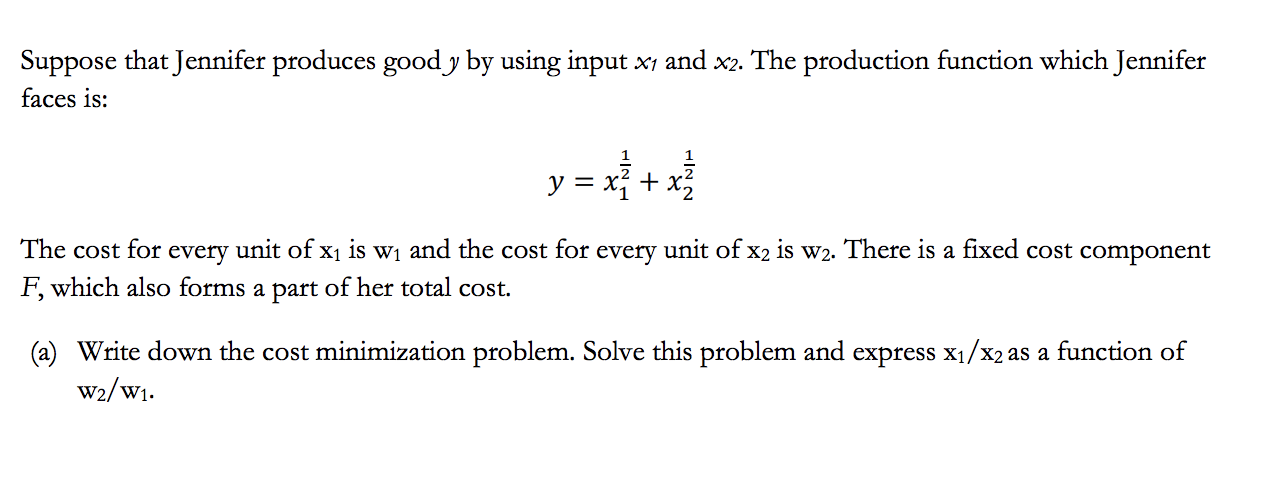Suppose that Jennifer produces good y by using input xi and x2. The production function which Jennifer faces is: y = x} + x3 The cost for every unit of xı is wi and the cost for every unit of x2 is w2. There is a fixed cost component F, which also forms a part of her total cost. (a) Write down the cost minimization problem. Solve this problem and express X1/X2 as a function of w2/w1.

• ### NEED ANSWERS OF PART (f,g,h,j) Problem 2 [21 marks] Consider a firm that uses two inputs. The quantity used of input 1...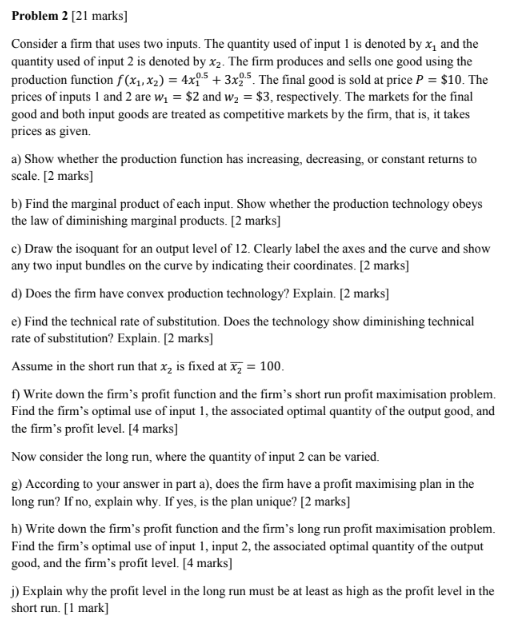NEED ANSWERS OF PART (f,g,h,j) Problem 2 [21 marks] Consider a firm that uses two inputs. The quantity used of input 1 is denoted by x, and the quantity used of input 2 is denoted by x2. The firm produces and sells one good using the production function f(x1, x2)-4x053x25. The final good is sold at price P \$10. The prices of inputs 1 and 2 are w\$2 and w2 \$3, respectively. The markets for the final good and both...

• ### Question 1: Cost Minimization and Cost Curves Suppose that Jennifer produces good y by using input...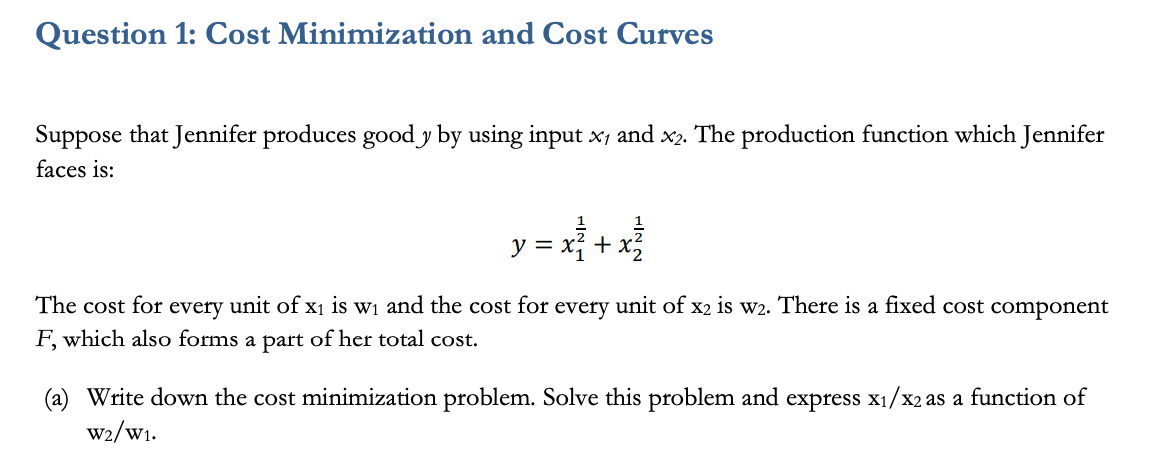Question 1: Cost Minimization and Cost Curves Suppose that Jennifer produces good y by using input xi and x2. The production function which Jennifer faces is: y = x} + x] The cost for every unit of xi is wi and the cost for every unit of x2 is w2. There is a fixed cost component F, which also forms a part of her total cost. (a) Write down the cost minimization problem. Solve this problem and express x1/x2 as...

• ### Question 3 (20 marks) Consider the optimization problem faced by a Monash University administrator who has the task...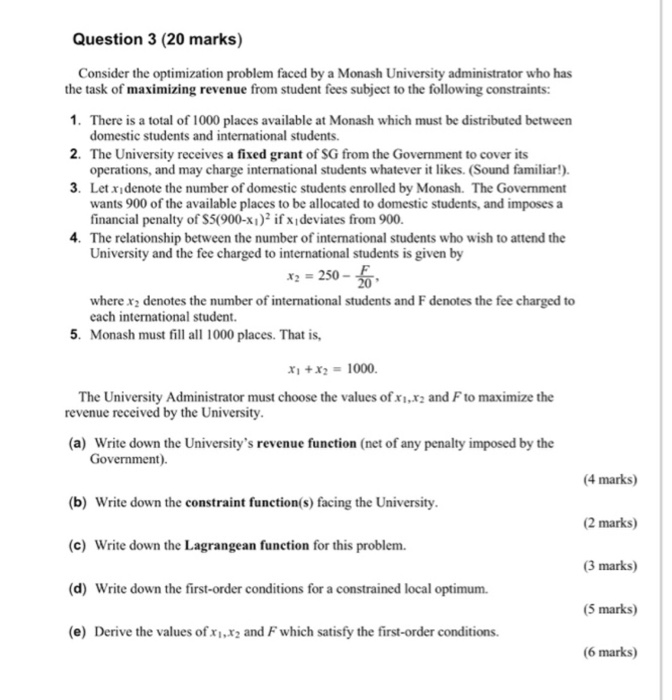Question 3 (20 marks) Consider the optimization problem faced by a Monash University administrator who has the task of maximizing revenue from student fees subject to the following constraints: 1. There is a total of 1000 places available at Monash which must be distributed between domestic students and international students 2、 The University receives a fixed grant of SG from the Government to cover its operations, and may charge international students whatever it likes. (Sound familiar!) 3. Letxdenote the number...

• ### NEED ALL ANSWERS PLEASE Problem 3 [24 marks] A competitive firm uses two inputs, capital (k) and labour (), to produce...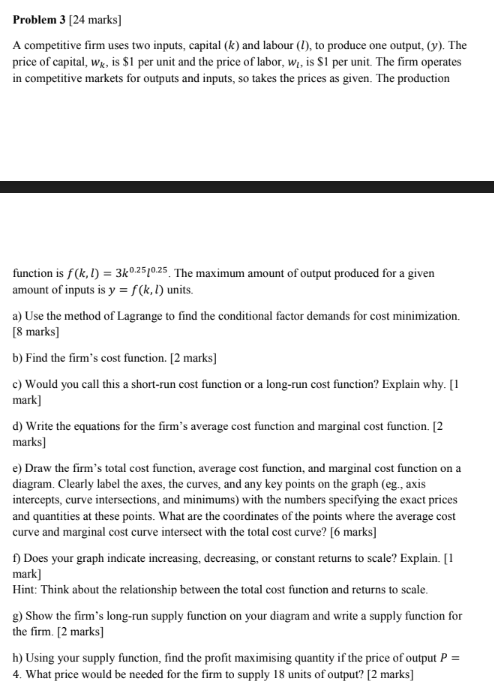NEED ALL ANSWERS PLEASE Problem 3 [24 marks] A competitive firm uses two inputs, capital (k) and labour (), to produce one output, (y). The price of capital, W, is S1 per unit and the price of labor, wi, is SI per unit. The firm operates in competitive markets for outputs and inputs, so takes the prices as given. The production function is f(k,l) 3k025/025. The maximum amount of output produced for a givern amount of inputs is y(k, l)...

• ### = Question 1: Cost Minimization and Cost Curves Suppose that Jennifer produces goody by using input...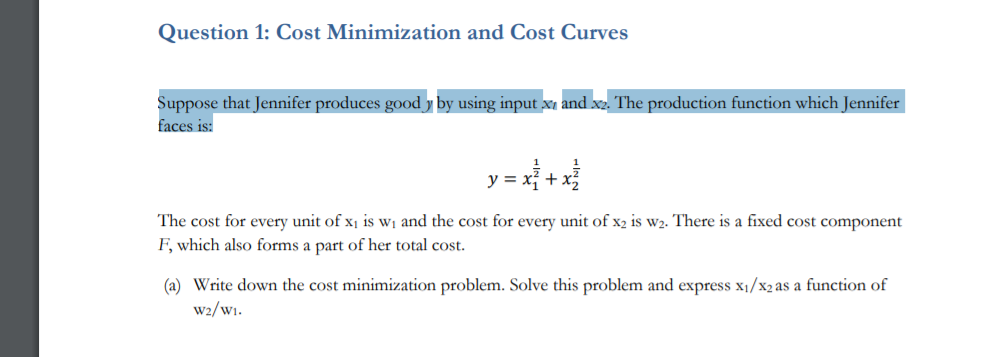= Question 1: Cost Minimization and Cost Curves Suppose that Jennifer produces goody by using input x and x2. The production function which Jennifer faces is: The cost for every unit of Xi is wi and the cost for every unit of x2 is w2. There is a fixed cost component F, which also forms a part of her total cost. (a) Write down the cost minimization problem. Solve this problem and express X1/X2 as a function of w2/wi. Microeconomics...

• ### Question 5 (20 marks) (a) i) A firm has production function y - f(x,z) -ax+Bz, for...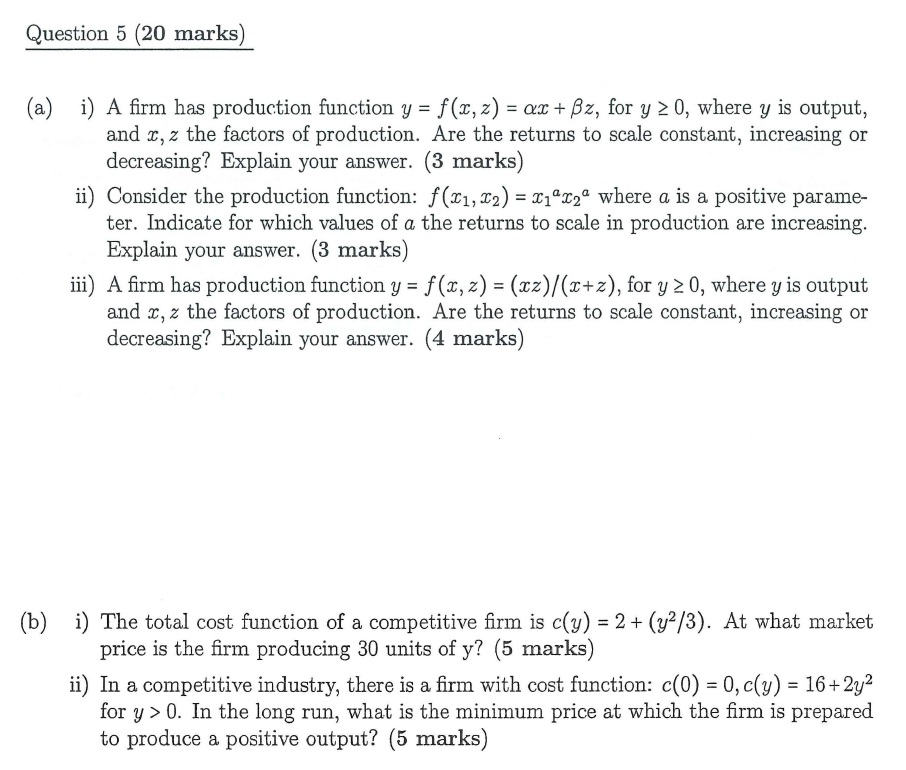Question 5 (20 marks) (a) i) A firm has production function y - f(x,z) -ax+Bz, for y 2 0, where y is output, and x, z the factors of production. Are the returns to scale constant, increasing or decreasing? Explain your answer. (3 marks) ii) Consider the production function: f(x1,2)2 where a is a positive parame- ter. Indicate for which values of a the returns to scale in production are increasing Explain your answer. (3 marks) iii) A firm has...

• ### Question 2: Firms Consider a firm that produces output Y from capital K and labour N...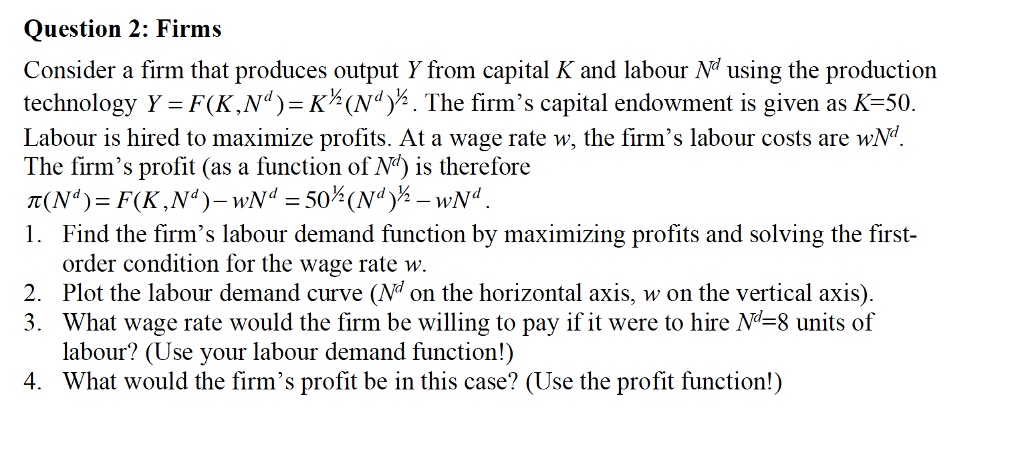Question 2: Firms Consider a firm that produces output Y from capital K and labour N using the production iechoolopy Y KNdThe f's capital endowcnt is piven as K 50 Labour is hired to maximize profits. At a wage rate w, the firm's labour costs are wN The firm's profit (as a function of N is therefore 1. Find the firm's labour demand function by maximizing profits and solving the fist order condition for the wage rate w. 2. Plot...

Need Online Homework Help?